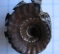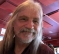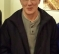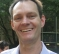## The size of an FIR filter for PDM-PCM conversionStarted by 3 years ago15 replieslatest reply 3 years ago1344 views

I have been trying to understand how the conversion from PDM to PCM happens so that I could interface with a microphone. I have been doing some research online and I found that the most common way to do this is to use an FIR filter and a decimator. I am having some trouble trying to determine the size of the FIR filter that I should use. Since in this case the FIR filter is using binary numbers, the output is affected by the 1’s density. If the coefficient of each tap is the same, then the number of possible values of each average is equal to the number of taps. If for example we have a 4 tap filter then 0101 would have the same results as for example 0110 since both values have the same density of 1’s. In this case the could be 5 different densities of 1 (0 – 1, 1-1, 2-1, 3-1, and 4-1) which can be stored in a 3 bit word. Using the same logic an FIR filter with 65534 taps would be needed to produce a 16 bit result. As already mentioned, in this case I am assuming that the coefficient are equal, example 1/N. I understand that the if the coefficients were not the same, then the results would be different because then the order of each bit would also make a difference but in this case, the order of the bits is irrelevant therefore a 1010 would yield the same results as 0110.

I hope that I have explained the problem that I am facing well. Can somebody please explain how a 16 bit resolution is obtained using FIR filter because to build a 65534 tap filter it becomes impractical.

[ - ]There are a couple guiding design principles that may be able to help you out.

The first is that the maximum output value of a FIR filter is the sum of the absolute values of the coefficients multiplied by the maximum input value.   If your PDM stream is only ones and zeros, then the maximum output value of the filter will be the sum of the absolute values of the coefficients. This also bounds the size of the accumulator required depending on what architecture you use for the FIR.

From this perspective the number of coefficients does not drive the size of the output word, but the coefficients themselves do.

The second is that the bandwidth reduction of the filter drives the actual precision increase.  For every 4:1 reduction in bandwidth, you can claim an actual increase in precision of one bit.  So if you want 16-bits of precision, the filtering needs to reduce the supported bandwidth by a factor of 64.   This is why decimation is also typically done during PDM to PCM conversion, because the bandwidth is being chopped way down and no longer needs the high PDM sample rate.

A filter with a narrow passband does usually require a large number of coefficients with a FIR filter, but there's no driving rule of how long it has to be.   If can design a filter with N coefficients that does what you need, there you are.   As someone else suggested, if you want to cascade filters, you can do that, too.   You can probably do a cascaded CIC filter and get the job done reasonably well from that perspective, although this is not something I've tried.

I hope that helps a bit.

[ - ]I have always used a CIC (cascaded Integrator comb) filter for this. It's also called a Haugenauer filter (my spelling might be off). You can Google it. Follow that with a typical FIR of length (Fs*atten(dB))/(transition BW * 22) and you're good to go.

If the final down conversion is a multiple of 2, you might be able to save processing by using several half rate filters as every other coef in said filter is 0. As with anything worth doing, how you implement this depends on your needs and resources. Best of luck.

P.S. There is a way to use a regular polyphase FIR and a state machine, but this is more complicated. I haven't tried that yet, but know it can be done.

[ - ]Something doesn't smell right here: Wouldn't a factor of 64 bandwidth reduction only gain you 3 bits of precision?

[ - ]Yeah, brain fart.  For 16 bits from 1 you need, what, 128k:1 reduction?

[ - ]unless you do noise shaping around the modulator.

16-bit audio converters don't do 128K x 48 kHz.

[ - ]This sent me down a rabbit-hole. For 1-bit -> 16-bit you'd need a 4^15 decimation rate - roughly 2 billion, so that's clearly not what's going on in a PDM->PCM conversion.

I think the key here is that PDM is noise shaped, so assuming flat noise across the entire bandwidth of the PDM sample rate is not a valid starting point. In the audio bandwidth (0-20kHz) the noise is actually more like 100dB down, so your conversion operation is not relying on the precision gain of decimation to increase its bitwidth. Instead it's more of a filtering operation that's removing the shaped noise out near the Nyquist rate of the PDM signal.

[ - ]Ahg, you made me go back and remember a bunch of things.

I wasn't assuming audio PDM as there are other PDM applications.  We used PDM as a single-wire interface to the old Qualcomm DDS devices to steer control loops.   The filter output fed a linear PCM -> PWM converter, essentially the carry-out of an integrator, that selected between the PIR registers in the DDS.  We got high-performance results with an upsampling rate in the several thousands, but the loop output to the integrator was typically only about eight bits or so, iirc.

It was possible to do the whole loop analysis very accurately, including estimating the DDS phase-noise sideband output levels and jitter performance, without making any assumptions of the noise shape other than the conversion was linear. Looking back now, I don't know how I got away with that, but results were always spot-on, so there was no peril in ignoring it.

Anyway, it sounds like it is getting covered reasonably well here for the OP, at least I hope so.

[ - ]i'm sorta a dummy.  what's "PDM"?  is that another name for "PWM"?

[ - ]Pulse Density Modulation.    Basically the amplitude is encoded in the density or duty cycle of a binary signal.

[ - ]so you LPF it, like you might with PWM, right?  are we talking about the output of a sigma-delta modulator?

[ - ]Robert-

It's like halftoning an image -- the idea is diffusing the error due to 1-bit digitization, shaping the noise and pushing the error into higher freq spectrum. With audio that needs an additional step of much higher sampling rate (e.g. 3 MHz), so error is out of the audible spectrum.

Why do this ?  Long cables for "digital microphones".

-Jeff

[ - ]Sorry rbj for this late reply, just snooping around and found this thread.  So, yes, a PDM is the output of a 1bit SDM, and consequently is noise shaped (I think you or someone asked that somewhere else).

[ - ]I would approach this as a frequency domain problem and come up with the frequency response that you want (using whatever filter type that suits you: FIR, IIR, a combination, multistage...). You probably want to keep 0-20k somewhat flat, while getting enough attenuation in 26k+ band, and all that depends on the PDM frequency spectrum you start with.

The 'conversion' from 1-bit to 16-bit will just happen automatically as you filter; as a matter of fact you'll probably have more than 16 after filtering...

[ - ]I would use a cascade of FIR filters. I implemented a microphone PDM to PCM converter in software as three cascaded FIR filters with a divide by 8, divide by 8 and finally divide by N to get to 48kHz to 8kHz. As a reference

gives a guide of how the filters I would use would be designed.

A particularly efficient implementation of the PDM decimation filter is to use multiple 8 bit lookup tables where you can input 8 bits of PDM to each one at a time and output one phase of the PDM which can be accumulated to get the decimated output.

If you are interested I can provide some slides on how this is achieved.

[ - ]